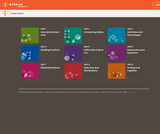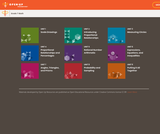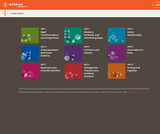Updating search results...# Open Up Resources

Open Up's mission is ito ncrease equity in education by making excellent, top-rated curricula freely available to districts.

3 affiliated resources

# Search Resources

View
Selected filters:Unrestricted Use
CC BY
Rating
0.0 stars

Student facing mathematics units. Covers area and surface area, ratios, dividing fractions, arithmetic in base ten, expressions and equations, rational numbers, and data sets/distributions.

Subject:
Mathematics
Material Type:
Unit of Study
Provider:
Open Up Resources
05/21/2019Unrestricted Use
CC BY
Rating
0.0 stars

Student-facing 7th grade math resources. Covers scale drawings, proportion, percentages, probability, expressions, and geometry.

Subject:
Mathematics
Material Type:
Unit of Study
Provider:
Open Up Resources
05/21/2019Unrestricted Use
CC BY
Rating
0.0 stars

Student-facing 8th grade math resources. Covers transformations & congruence, slope, similarity, linear relationships, associations in data, volume, functions, scientific notation, the pythagorean theorem and irrational numbers.

Subject:
Mathematics
Material Type:
Unit of Study
Provider:
Open Up Resources